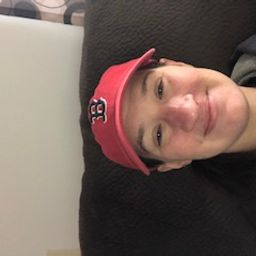💬 👋 We’re always here. Join our Discord to connect with other students 24/7, any time, night or day.Join Here!# Julie S.

Syracuse University

## Biography

Hi! My name is Julie and I have been a high school math teacher for 11 years. I have taught almost every math class offered at the high school level, for example Algebra 1, Geometry, Algebra 2, Trigonometry, PreCalculus, and AP Calculus. I enjoy helping students learn new mathematical concepts, so feel free to reach out if you have any questions.

## Education

BA Mathematics and Mathematics Education
Syracuse University

## Topics Covered

Functions
Functions
Rational Functions
Exponents and Polynomials
Polynomials
Graphs and Statistics
Equations and Inequalities
Linear Functions
Systems of Equations and Inequalities
Algebra Topics That are Reviewed at the Start of the Semester
The Integers
An Introduction to Geometry
Introduction to Algebra
Introduction to Conic Sections
Introduction to Conic Section
Series
Introduction to Sequences and Series
The Language of Algebra
Introduction to Sequences and Series
Whole which of Numbers
Trigonometry
Introduction to Trigonometry
Exponential and Logarithmic Functions
Fractions and Mixed Numbers
Ratio, Proportion, and Measurement
Graph Linear Functions
Write Linear Equations
Linear Equations and Functions
Matrices and Determinants
Linear Equations and Inequalities
Complex Numbers
Right Triangles
Circles
Decimals
Introduction to Matrices
Introduction to Combinatorics and Probability
Sequences
Factoring Polynomials
Congruent Triangles
Solve Linear Inequalities
Introduction to Combinatorics and Probability
Introduction to Vectors
Area and Perimeter
Relationships Within Triangles
Logic
Parametric Equations
Polar Coordinates
Geometric Proof
Angles
Parallel and Perpendicular lines
Geometry Basics